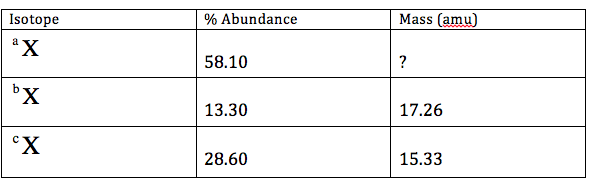# Problem: The element X has three naturally occuring isotopes;  aX, bX, and cX, where a, b, and c represent the mass number. The masses (amu),except aX, and the percent abundances of the isotopes are given in the table below. The average atomic mass of X is 17.20 amu. What is the mass of aX in amu? a. 16.60 b. 16.67 c. 19.01 d. 18.11 e. 9.99

85% (7 ratings)
###### Problem Details

The element X has three naturally occuring isotopes;  aX, bX, and cX, where a, b, and c represent the mass number. The masses (amu),except aX, and the percent abundances of the isotopes are given in the table below. The average atomic mass of X is 17.20 amu. What is the mass of aX in amu?

a. 16.60

b. 16.67

c. 19.01

d. 18.11

e. 9.99What scientific concept do you need to know in order to solve this problem?

Our tutors have indicated that to solve this problem you will need to apply the Atomic Mass concept. You can view video lessons to learn Atomic Mass. Or if you need more Atomic Mass practice, you can also practice Atomic Mass practice problems.

What is the difficulty of this problem?

Our tutors rated the difficulty ofThe element X has three naturally occuring isotopes;  aX, bX...as high difficulty.

How long does this problem take to solve?

Our expert Chemistry tutor, Dasha took 7 minutes and 51 seconds to solve this problem. You can follow their steps in the video explanation above.

What professor is this problem relevant for?

Based on our data, we think this problem is relevant for Professor Guo's class at GSU.